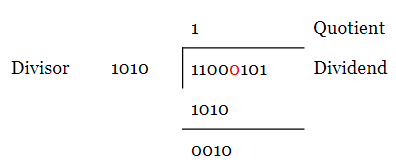Basic Binary Division: The Algorithm and the VHDL CodeBased on this algorithm, we’ll derive a block diagram for the circuit implementation of binary division. Finally, we’ll look at the ASMD (Algorithmic State Machine with a Data path) chart and the VHDL code of this binary divider. Consider dividing 11000101 by 1010.